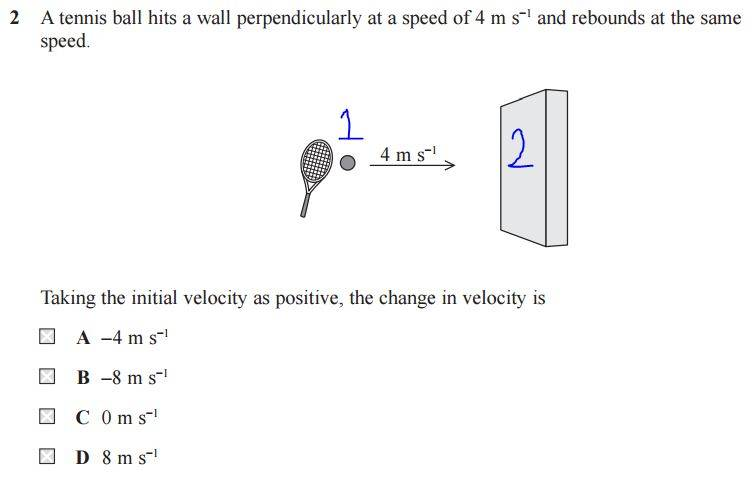# Change in Velocity Question

## Homework Statement## The Attempt at a Solution

[/B]
Assuming the right direction is positive, it hits the wall and reaches point 2, so to get back to point 1, it's in a negative direction, so it should be MINUS 4m so option A but the correct answer is B??

Or is it to do with total displacement? In which case would that be zero then? Since it hits the wall then comes back to point 1 anyway.

Chestermiller
Mentor

## Homework Statement

View attachment 111191

## The Attempt at a Solution

[/B]
Assuming the right direction is positive, it hits the wall and reaches point 2, so to get back to point 1, it's in a negative direction, so it should be MINUS 4m so option A but the correct answer is B??

Or is it to do with total displacement? In which case would that be zero then? Since it hits the wall then comes back to point 1 anyway.
Why do you feel that the total displacement has anything to do with this?

gneill
Mentor
You're looking for the change in velocity. That implies that there's an initial velocity and a final velocity, say vi and vf. Mathematically, how would you determine the change in velocity given those two variables?

Edit: FYI, I changed the thread title to make it a bit less generic.

You're looking for the change in velocity. That implies that there's an initial velocity and a final velocity, say vi and vf. Mathematically, how would you determine the change in velocity given those two variables?

Edit: FYI, I changed the thread title to make it a bit less generic.

Okay, with that line of thinking, the initial velocity is 4 and if the ball rebounds at the same speed (but in the opposite direction) isn't the final velocity also (-)4? so change in velocity would be 0??

jbriggs444
Homework Helper
Okay, with that line of thinking, the initial velocity is 4 and if the ball rebounds at the same speed (but in the opposite direction) isn't the final velocity also (-)4? so change in velocity would be 0??
Is -4 the same as +4?

Is -4 the same as +4?

okay where am I going wrong here?

the ball starts at point 1 with velocity of 4......it hits the wall and rebounds, also with velocity of 4 but since it's in the opposite direction, it's minus 4......so change in velocity is 4 + (-4) = zero??

gneill
Mentor
okay where am I going wrong here?

the ball starts at point 1 with velocity of 4......it hits the wall and rebounds, also with velocity of 4 but since it's in the opposite direction, it's minus 4......so change in velocity is 4 + (-4) = zero??
Do you add things together to find the change (difference)?

Previously I suggested that you write down the formula for determining the change in a quantity given initial and final values of that quantity. Did you do that?

Perhaps you need an example that doesn't involve a negative value to get the idea straight first. Suppose the initial velocity was 2 m/s and the final velocity was 8 m/s. What would be the change in velocity? How would you write that mathematically?

Do you add things together to find the change (difference)?

Previously I suggested that you write down the formula for determining the change in a quantity given initial and final values of that quantity. Did you do that?

Perhaps you need an example that doesn't involve a negative value to get the idea straight first. Suppose the initial velocity was 2 m/s and the final velocity was 8 m/s. What would be the change in velocity? How would you write that mathematically?

so change in velocity is final velocity minus initial velocity = 8 - 2 = 6m/s ?

which means in my example, final velocity - initial velocity = -4 - 4 = -8m/s

but I don't get what the -8m/s represents? that is moved in the negative direction at 8m/s??

gneill
Mentor
so change in velocity is final velocity minus initial velocity = 8 - 2 = 6m/s ?

which means in my example, final velocity - initial velocity = -4 - 4 = -8m/s

but I don't get what the -8m/s represents? that is moved in the negative direction at 8m/s??
It means that the ball's velocity changed by -8 m/s.

Velocity is a vector. You chose the coordinate system (direction of the +x axis) when you declared the initial velocity to be positive. Both the initial and final velocities are vector quantities. When you add or subtract vectors, the result is also a vector. Every vector has both a magnitude and a direction. The magnitude of the change in velocity was 8 m/s and the change occurred in the -x direction.